Note: This is a working paper which will be expanded/updated frequently. One way to think about this paper is as an update of De Leeuw (2014), using simpler and more direct majorization methods. The directory deleeuwpdx.net/pubfolders/rstress has a pdf copy of this article, the complete Rmd file that includes all code chunks, and R files with the code.

# 1 Problem

Define the multidimensional scaling (MDS) loss function $\begin{equation} \sigma_r(x)=\sum_{i=1}^nw_i(\delta_i-(x'A_ix)^r)^2,\label{E:rstress} \end{equation}$

with $$r>0$$ and the $$A_i$$ positive semi-definite. The $$w_i$$ are positive weights, the $$\delta_i$$ are non-negative dissimilarities. We call this rStress. Special cases are stress (Kruskal 1964) for $$r=\frac12$$, sstress (Takane, Young, and De Leeuw 1977) for $$r=1$$, and the loss function used in MULTISCALE (Ramsay 1977) for $$r\rightarrow 0$$.

Usually MDS is formulated uses Euclidean distances $$d_{j\ell}(X)$$ between points $$j$$ and $$\ell$$, which are rows of an $$n\times p$$ configuration matrix $$X$$. This fits into our formulation by setting $$x=\mathbf{vec}(X)$$ and by setting the $$A_i$$ to $$np\times np$$ matrices of the form $$I_p\otimes E_{j\ell}$$, where the matrix $$E_{j\ell}$$ has elements $$(j,j)$$ and $$(\ell,\ell)$$ equal to $$+1$$ and elements $$(j,\ell)$$ and $$(\ell,j)$$ equal to $$-1$$. Thus the $$A_i$$ are direct sums of $$p$$ copies of the $$E_{j\ell}$$. Now $$x'A_ix=d_{j\ell}^2(X)$$.

The problem we are trying to solve is to find an algorithm to minimize $$\sigma_r$$ over $$x$$ in $$\mathbb{R}^m$$ for all values of $$r>0$$. It is understood that parts of the algorithm may be different for different values of $$r$$.

# 2 Minorization and Majorization

We will design a convergent iterative majorization algorithm. Briefly this means constructing for each $$r>0$$ a majorization function $$\gamma_r$$ on $$\mathbb{R}^m\otimes\mathbb{R}^m$$ such that $$\sigma_r(x)\leq\gamma_r(x,y)$$ for all $$x$$ and $$y$$ and such that $$\sigma_r(x)=\gamma_r(x,x)$$ for all $$x$$. One step of the iterative algorithm is $x^{(k+1)}=\text{arg}\min_x\gamma_r(x,x^{(k)}),$ unless $$x^{(k)}$$ already minimizes $$\gamma_r(x,x^{(k)})$$, in which case we stop. If we do not stop we have the sandwich inequality $\sigma_r(x^{(k+1)})\leq\gamma_r(x^{(k+1)},x^{(k)})<\gamma_r(x^{(k)},x^{(k)})=\sigma_r(x^{(k)}).$ Thus majorization algorithms exhibit monotone convergence of loss function values.

The history of majorization algorithms is complicated. They were first developed in the specific contexts of step-size estimation in descent algorothms (Ortega and Rheinboldt 1970), maximum likelihood estimation with missing data (Dempster, Laird, and Rubin 1977), and multidimensional scaling (De Leeuw 1977). Subsequently they were presented as a general class of optimization methods, and as a special case of block relaxation and augmentation methods, by De Leeuw (1994), see also Heiser (1995). The material in De Leeuw (1994) is (slowly, slowly) expanded into an e-book, with one part on block relaxation, augmentation and alternating least squares (“Homogeneity Analysis” 2015), one part on majorization (De Leeuw 2015a), and one part on mathematical background (De Leeuw 2015b). There have been many applicatons of the general majorization principle by The Dutch School in psychometrics, which includes Ten Berge, Kiers, Heiser, Meulman, Groenen, and Vermunt.

Systematic use of majorization in statistics started with Lange, Hunter, and Yang (2000). There have been many further reviews, developments, and applications by Ken Lange and his co-workers. Theyo use the name MM Algorithms, where MM stands for either majorization-minimization or minorization-maximization. Lange (2013) has a very nice chapter on the MM algorithm, and he is currently working on an MM methods book.

# 3 Use of Homogeneity

We start by exploring the obvious fact that $\min_x\sigma_r(x)=\min_{\theta\geq 0}\min_{x'x=1}\sigma(\theta x).$ Let us introduce some notation to simplify the discussion. Without loss of generality we assume the dissimilarities are scaled by $\sum_{i=1}^nw_i\delta_i^2=1.$ Next, it is convenient to define $\rho_r(x):=\sum_{i=1}^nw_i\delta_i(x'A_ix)^r,$ and $\eta_r(x):=\sum_{i=1}^nw_i(x'A_ix)^{2r},$ so that $\begin{equation} \sigma_r(x)=1-2\rho_r(x)+\eta_r(x).\label{E:expand} \end{equation}$ Now $\begin{equation} \sigma_r(\theta x)=1-2\theta^{2r}\rho_r(x)+\theta^{4r}\eta_r(x).\label{E:homo} \end{equation}$ Thus, as in De Leeuw (1977), thinking of rStress as a function of both $$x$$ and $$\theta$$, $\begin{equation} \min_\theta\sigma_r(\theta, x)=1-\frac{\rho_r^2(x)}{\eta_r(x)},\label{E:theta} \end{equation}$ where the minimium is attained at $\begin{equation} \theta^{2r}=\frac{\rho_r(x)}{\eta_r(x)}.\label{E:optt} \end{equation}$

Thus minimizing $$\sigma_r$$ can be done by maximizing the ratio in $$\eqref{E:theta}$$ over $$x'x=1$$. This is used in the majorization method proposed by De Leeuw (2014), by combining Dinkelbach (1967) and quadratic majorization.

The approach of De Leeuw (2014) is somewhat cumbersome, because we first use homogeneity to reduce the MDS problem to a fractional programming problem, and then we use Dinkelbach to get rid of the fractional objective funcion again. Moreover one of the special cases requires us to solve a modified eigenvalue problem of the type discussed, for example, by Hager (2001) in each iteration.

In this paper we combine majorization with alternating least squares, working directly with (3). Thus we think of rStress as a function of both $$\theta$$ and $$x$$, and we alternate minimization over $$\theta$$ for fixed $$x$$ and over $$x$$ for fixed $$\theta$$. Thus $\begin{split} \theta^{(k)}&=\text{arg}\min_\theta\sigma_r(\theta, x^{(k)}),\\ x^{(k+1)}&=\text{arg}\min_{x'x=1}\sigma_r(\theta^{(k)},x). \end{split}$ The first step is trivially solved using $$\eqref{E:optt}$$. Updating $$x$$, however, is far from trivial and needs the majorization machinery we will develop in this paper. Since updating $$x$$ is iterative, we have to choose how many majorization steps to take for updating $$x$$, before going to the next update for $$\theta$$.

Thus the main focus of the paper will be a majorization method for minimizing $\begin{equation} \sigma_r(x,\alpha):=1-2\alpha\rho_r(x)+\alpha^2\eta_r(x)\label{E:lambda} \end{equation}$

over $$x'x=1$$ with $$\alpha:=\theta^{2r}$$ fixed at its current value. It is clear from $$\eqref{E:lambda}$$ that we can find a majorization of $$\sigma_r$$ by combining a minorization of $$\rho_r$$ and a majorization of $$\eta_r$$.

# 4 Powers of Quadratic Forms

We start with some lemmas we will need to construct our minorizations and majorizations.

Lemma 4.1: $$f_r(x):=(x'Ax)^r$$ is convex on $$x'Ax>0$$ if and only if $$r\geq\frac12$$.

Proof: The first and second derivative are $\mathcal{D}f_r(x)=2r(x'Ax)^{r-1}Ax,$ and $\mathcal{D}^2f_r(x)=2r(x'Ax)^{r-1}\left(A+2(r-1)\frac{Axx'A}{x'Ax}\right).$ The matrix $$H_r(x):= A+ 2(r-1)\frac{Axx'A}{x'Ax}$$ is psd for $$r=\frac12$$, and its eigenvalues increase with $$r$$. Thus it is psd for all $$r\geq\frac12$$.

Also, if $$0<r<\frac12$$ then, by Sylvester’s Law of Inertia, $$\mathcal{D}^2f_r(x)$$ has precisely one negative eigenvalue, as well as $$\mathbf{rank}(A)-1$$ positive eigenvalues, and $$n-\mathbf{rank}(A)$$ zero eigenvalues. Thus in this case $$f_r$$ is not convex (and not concave either). QED

By the way, we can use the definition of convexity to show lemma 4.1 is true for all $$x$$, not just for $$x$$ with $$x'Ax>0$$.

Lemma 4.2: If $$r\geq\frac12$$ then $f_r(x)\geq(1-2r)f_r(y)+2rf_{r-1}(y)y'Ax.$

Proof: Follows from the fact that $$f_r$$ is convex, i.e. above each of its tangents. QED

Now write $$\overline{\lambda}(X)$$ or $$\overline{\lambda}_X$$ for the largest eigenvalue of a matrix $$X$$, and $$\underline{\lambda}(X)$$ or $$\underline{\lambda}_X$$ for the smallest eigenvalue. Note that if $$A=I\otimes E_{j\ell}$$ then $$\overline{\lambda}_A=2$$.

Lemma 4.3: If $$r\geq 1$$ then $\overline{\lambda}(\mathcal{D}^2f_r(x))\leq 2r(2r-1)\overline{\lambda}^r_A\ (x'x)^{r-1}.$ If $$x'x\leq 1$$ then $\overline{\lambda}(\mathcal{D}^2f_r(x))\leq 2r(2r-1)\overline{\lambda}^r_A.$ Proof: If $$r\geq 1$$, then $u'H_r(x)u=u'Au+2(r-1)\frac{(u'Ax)^2}{x'Ax}\leq (2r-1)u'Au.$ Thus $\overline{\lambda}(H_r(x))\leq (2r-1)\overline{\lambda}_A,$ and $\overline{\lambda}(\mathcal{D}^2f_r(x))\leq 2r(2r-1)\overline{\lambda}_A(x'Ax)^{r-1} \leq 2r(2r-1)\overline{\lambda}^r_A\ (x'x)^{r-1}.$ Finally, if $$x'x\leq 1$$ then $$(x'x)^{r-1}\leq 1$$. QED

Lemma 4.4: If $$0<r\leq 1$$ then $f_r(x)\leq (1-r)f_r(y)+rf_{r-1}(y)x'Ax.$

Proof: If $$r\leq 1$$ then $$(x'Ax)^r$$ is concave in $$x'Ax$$, although not in $$x$$. Thus $$f_r$$ is below its tangents, and $f_r(x)\leq f_r(y)+r(y'Ay)^{r-1}(x'Ax-y'Ay),$ which simplifies to the required result. QED

Lemma 4.5: If $$0<r\leq 1$$ and $$x'x=y'y=1$$ then $f_r(x)\leq(1-2r)f_r(y)+2rf_{r-1}(y)(x'(A-\overline{\lambda}_A I)y+\overline{\lambda}_A).$

Proof: From $x'Ax=y'Ay+2y'A(x-y)+(x-y)'A(x-y)\leq -y'Ay+2y'Ax+\overline{\lambda}_A(x-y)'(x-y)$ we see that if $$x'x=y'y=1$$ that $x'Ax\leq 2x'(A-\overline{\lambda}_A I)y+2\overline{\lambda}_A-y'Ay.$ Substitute this in lemma 4.4 and simplify. QED

Lemma 4.6: If $$0<r<\frac12$$ then $\underline{\lambda}(\mathcal{D}^2f_r(x))\geq 2r(2r-1)\overline{\lambda}^r_A(x'x)^{r-1}.$ If $$x'x\leq 1$$ then $\underline{\lambda}(\mathcal{D}^2f_r(x))\geq 2r(2r-1)\overline{\lambda}^r_A.$

Proof: We have $\frac{(u'Ax)^2}{x'Ax}\leq u'Au$ as before. Thus $u'H(x)u\geq(2r-1)u'Au\geq(2r-1)\overline{\lambda}_Au'u.$ The result follows because in addition $$x'Ax\leq\overline{\lambda}_A x'x$$, and consequently $$(x'Ax)^{r-1}\geq\overline{\lambda}_A^{r-1}(x'x)^{r-1}$$. Moreover if $$x'x\leq 1$$ then $$(x'x)^{r-1}\geq 1$$. QED

The following lemma, defining a type of uniform quadratic majorization (De Leeuw and Lange 2009), is an additional useful tool. Because we work on the unit sphere, the quadratic majorization actually becomes linear. This relies heavily on the fact that if $$x'x=y'y=1$$ and $$z$$ is on the segment $$[x,y]$$ connecting $$x$$ and $$y$$, then $$z'z\leq 1$$.

Lemma 4.7: Suppose $$\phi$$ is any homogeneous function of degree $$s$$, $$x'x=y'y=1$$ and $$\overline{\lambda}(\mathcal{D}^2\phi(z))\leq\kappa$$ for all $$z\in[x,y]$$. Then $\phi(x)\leq(1-s)\phi(y)+\kappa+x'(\mathcal{D}\phi(y)-\kappa y).$ In the same way, if $$\underline{\lambda}(\mathcal{D}^2\phi(z))\geq\kappa$$ for all $$z\in[x,y]$$ we have $\phi(x)\geq(1-s)\phi(y)+\kappa+x'(\mathcal{D}\phi(y)-\kappa y).$

Proof: We only prove the first part. The proof of the second part goes the same. By Taylor’s theorem we have for all $$x$$ and $$y$$ $\phi(x)\leq\phi(y)+(x-y)'\mathcal{D}\phi(y)+\frac12\kappa(x-y)'(x-y),$ which simplifies to the stated result if $$x'x=y'y=1$$ and $$\phi$$ is homogeneous of degree $$s$$. QED

# 5 Majorizing rStress

We will now use the inequalities from the lemmas in the previous section to derive linear minorizations of $$\sigma_r$$, by combining majorization of $$\eta_r$$ and minorization of $$\rho_r$$. Our majorizations are in the form $$f(x)\leq x'g(y)+\epsilon(y)$$, where both $$g$$ and $$\epsilon$$ are functions which do not depend on $$x$$, only on $$y$$. Since the precise form of $$\epsilon$$ has no influence on the iterative algorithm we usually leave it unspecified, so in different formulas $$\epsilon$$ can refer to different funcions. Clearly the majorization algorithm will have the form $\begin{equation} x^{(k+1)}=-\frac{g(x^{(k)})}{\|g(x^{(k)})\|}.\label{E:major} \end{equation}$ For the two key results of this paper we need to define matrices $\begin{equation} B_r(y):=\sum_{i=1}^n w_i\delta_i(y'A_iy)^{r-1}A_i,\label{E:bdef} \end{equation}$ and $\begin{equation} C_r(y):=\sum_{i=1}^n w_i(y'A_iy)^{2r-1}A_i,\label{E:cdef} \end{equation}$ and scalars $\begin{equation} \beta_r:=(2r-1)\sum_{i=1}^n w_i\delta_i\overline{\lambda}^r(A_i),\label{E:bedef} \end{equation}$ and $\begin{equation} \gamma_r:=\sum_{i=1}^n w_i(y'A_iy)^{2r-1}\overline{\lambda}(A_i),\label{E:gadef} \end{equation}$ and $\begin{equation} \delta_r:=(4r-1)\sum_{i=1}^n w_i\overline{\lambda}^{2r}(A_i).\label{E:dedef} \end{equation}$

Note that $$\delta_r=\beta_{2r}$$. Also $$y'B_r(y)y=\rho_r(y)$$ and $$y'C_r(y)y=\eta_r(y)$$.

Theorem 5.1: If $$0<r\leq\frac12$$ then $$\eqref{E:major}$$ with $\begin{equation} g(y)=-\{(B_r(y)-\beta_rI)-\alpha(C_r(y)-\gamma_rI)\}y\label{E:gsmall} \end{equation}$

is a convergent majorization algorithm.

Proof: To majorize $$\eta_r$$ we use lemma 4.5. After some calculation we find the linear majorization $\eta_r(x)\leq 4rx'(C_r(y)-\gamma_rI)y+\epsilon(y),$ where $$\epsilon$$ is some function which only depends on $$y$$ and not on $$x$$.

To minorize $$\rho_r$$ we use lemma 4.7 with $$\phi$$ equal to $$f_r$$. Note that $$f_r$$ is homogeneous of order $$2r$$. Thus $(x'A_ix)^r\geq(1-2r)(y'A_iy)^r+\kappa_i+x'(2r(y'A_iy)^{r-1}A_i-\kappa_i I)y$ By lemma 4.6 we have $$\kappa_i=2r(2r-1)\overline{\lambda}^r(A_i)$$. Thus $\rho_r(x)\geq 2rx'(B_r(y)-\beta_r I)y + \epsilon(y).$

By combining the results for $$\rho_r$$ and $$\eta_r$$ we see that $\sigma_r(x)\leq 4\alpha rx'g(y)+\epsilon(y)$ with $$g(y)$$ defined by $$\eqref{E:gsmall}$$. QED

Theorem 5.2: If $$r\geq\frac12$$ then $$\eqref{E:major}$$ with $\begin{equation} g(y):=-\{(B_r(y)-\alpha(C_r(y)-\delta_r I)\}y\label{E:glarge} \end{equation}$

is a convergent majorization algorithm.

Proof: We can use lemma 4.2 to get $\rho_r(x)\geq 2rx'B_r(y)y+\epsilon(y).$

Because $$\eta_r$$ is homogeneous of order $$4r$$ we see from lemma 4.7 that $\eta_r(x)\leq 4rx'(C_r(y)-\delta_r I)y+\epsilon(y).$

By combining the results for $$\rho_r$$ and $$\eta_r$$ we see that $\sigma_r(x)\leq 4r\alpha x'g(y)+\epsilon(y)$ with $$g(y)$$ defined by $$\eqref{E:glarge}$$. QED

# 6 Special Considerations

If $$r=\frac12$$ we have $$\beta_r=0$$, and $B_r(y)=\sum_{i=1}^n w_i\frac{\delta_i}{\sqrt{y'A_iy}}A_i,$ and $C_r(y)=\sum_{i=1}^n w_iA_i.$ These are precisely the matrices used in the SMACOF algorithm of De Leeuw (1977), implemented in De Leeuw and Mair (2009), which is $x^{(k+1)}=C_r^+(x^{(k)})B_r(x^{(k)})x^{(k)},$ where $$C_r^+$$ is the Moore-Penrose inverse. Also note that if $$r=\frac12$$ then $$\gamma_r=\delta_r$$ and the algorithms of theorems 5.1 and 5.2 are the same.

The case $$r\rightarrow 0$$ also requires some special attention. Define $\sigma_0(x):=\sum_{i=1}^nw_i(\log\delta_i-\log x'A_ix)^2.$ Note that $\sum_{i=1}^nw_i(\log\delta_i^r-\log (x'A_ix)^r)^2=r^2\sigma_0(x),$ which means that minimizing $$\sum_{i=1}^nw_i(\log\delta_i^r-\log (x'A_ix)^r)^2$$ for any $$r>0$$ can be done by minimizing $$\sigma_0$$.

Now use $\log x=\lim_{r\rightarrow 0}\frac{x^r-1}{r}.$ Thus, for small $$r$$, $\log\delta_{i}-\log x'A_ix\approx\frac{\delta_i^r-(x'A_ix)^r}{r},$ and $\sigma_0(x)\approx \frac{1}{r^2}\sum_{i=1}^n w_i(\delta_i^r-(x'A_ix)^r)^2.$ On the other hand, if $$\delta_i\approx x'A_ix$$ then for any $$r$$ we have the approximation $\log (x'A_ix)^r\approx\log\delta_i^r+\frac{1}{\delta_i^r}((x'A_ix)^r-\delta_i^r),$ so that $\sigma_0(x)\approx \sum_{i=1}^n \frac{w_i}{\delta_i^{2r}}(\delta_i^r-(x'A_ix)^r)^2.$ Both approximatations to $$\sigma_0$$ can be minimized by our iterative majorization algorithm for $$r<\frac12$$.

Note that our definition of rStress compares dissimilarities with powers of Euclidean distances. Alternatively, we can compare powers of dissimilarities with corresponding powers of Euclidean distances, i.e. we can define another rStress as $\begin{equation} \tilde\sigma_r(x):=\sum_{i=1}^n w_i((\delta_i^2)^r-(x'A_ix)^r)^2.\label{E:tilde} \end{equation}$

This is similar to our approach in the limiting case $$r\rightarrow 0$$. We do not need an additional algorithm to minimize $$\tilde\sigma_r$$, because we can just input the $$(\delta_i^2)^r$$ instead of $$\delta_i$$ in our previous majorization method.

The difference between $$\sigma_r$$ and $$\tilde\sigma_r$$ disappears, of course, if we make the algorithm nonmetric. In that case we alternate minimization over $$x$$ with minimization over monotone transformations of $$\delta$$ (or of $$\delta^r$$, which is of course the same thing). We could easily add a monotone regression step to our alternating least squares algorithm.

# 7 Examples

## 7.1 Artificial

Let’s start with a small example that shows the flow of the algorithm. We perform one iteration and write out the intermediary results in detail.

Suppose $$n=3$$, the weights $$w$$ are all one, and the dissimilarities are 1, 2, 3. The matrices $$A$$ are

##      [,1] [,2] [,3] [,4]
## [1,]    1    1   -1   -1
## [2,]    1    1   -1   -1
## [3,]   -1   -1    1    1
## [4,]   -1   -1    1    1
##      [,1] [,2] [,3] [,4]
## [1,]    1   -1   -1    1
## [2,]   -1    1    1   -1
## [3,]   -1    1    1   -1
## [4,]    1   -1   -1    1
##      [,1] [,2] [,3] [,4]
## [1,]    1   -1    1   -1
## [2,]   -1    1   -1    1
## [3,]    1   -1    1   -1
## [4,]   -1    1   -1    1

The largest eigenvalues of all three $$A$$ matrices are equal to 4. Thus $$\delta_r$$ of $$\eqref{}$$ is 144.

Let’s start with $$x$$ four random normals. We want to minimize rStress for $$r=1$$, i.e. sStress.

##  3.4514035 0.2191467 0.0485130

At the start sStress is 17.892093. Also $$\rho$$ is 4.0352358 and $$\eta$$ is 11.9625647. This means $$\alpha$$ is 0.337322 and the minimum of rStress over $$\alpha$$ is 12.6388263.

We now compute the matrices $$B_r$$ and $$C_r$$ at $$x$$.

For $$B_r$$ we have

##      [,1] [,2] [,3] [,4]
## [1,]    6   -4    0   -2
## [2,]   -4    6   -2    0
## [3,]    0   -2    6   -4
## [4,]   -2    0   -4    6

and for $$C_r$$

##           [,1]      [,2]      [,3]      [,4]
## [1,]  3.719063  3.183744 -3.622037 -3.280770
## [2,]  3.183744  3.719063 -3.280770 -3.622037
## [3,] -3.622037 -3.280770  3.719063  3.183744
## [4,] -3.280770 -3.622037  3.183744  3.719063

Also, $$\delta_r$$ is equal to 144 and thus

Now $$g$$ is

##   9.408613 11.604293 -1.162210 -7.853545

and the new $$x$$ is

##  -0.55613830 -0.68592383  0.06869765  0.46421904

With this $$x$$ sStress is 12.4979159. We now go back, compute a new $$\alpha$$, and so on.

## 7.2 Dutch Political Parties

De Gruijter (1967) collected dissimilarity measures for nine Dutch political parties.

##       KVP PvdA  VVD  ARP  CHU  CPN  PSP   BP
## PvdA 5.63
## VVD  5.27 6.72
## ARP  4.60 5.64 5.46
## CHU  4.80 6.22 4.97 3.20
## CPN  7.54 5.12 8.13 7.84 7.80
## PSP  6.73 4.59 7.55 6.73 7.08 4.08
## BP   7.18 7.22 6.90 7.28 6.96 6.34 6.88
## D66  6.17 5.47 4.67 6.13 6.04 7.42 6.36 7.36
The solutions for 0.1, 0.25, 0.5, 0.75, 1, 2 are given in figure 1.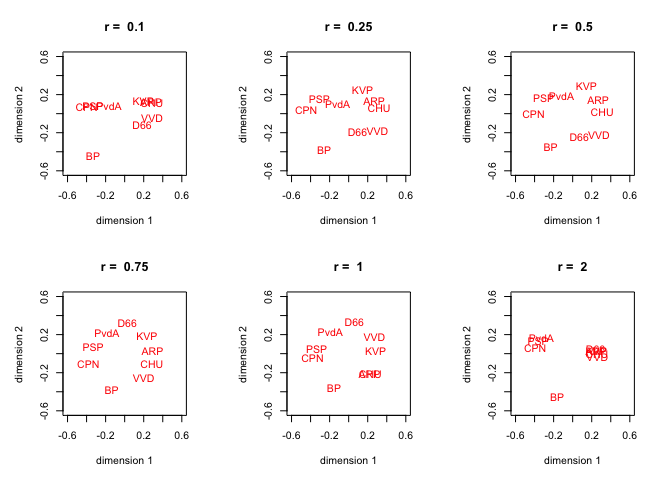Figure 1: De Gruijter Data: Separate Configurations

The basic structure for all solutions is the same, we see the familiar horseshoe, in which the left-right scale is bended so that extreme left (CPN) and extreme right (BP) are close. This is perhaps most clear for r = .75. If r becomes large, then we see increasing clustering.

We also give all six solutions plotted on top of each other (after Procrustus matching) in figure 9. It shows that the two extreme parties are stable over solutions, but the middle is more variable.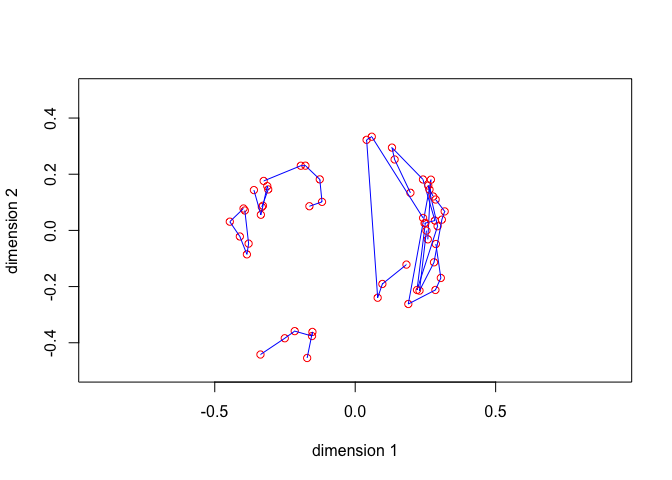Figure 2: De Gruijter Data: Joint Configuration

Plotting rStress in figure 3 as a function of r shows, somewhat surprisingly perhaps, an increasing function. Because the data are average dissimilarities, it is likely there is a fairly large additive constant, and additive constants correspond with lower values of r.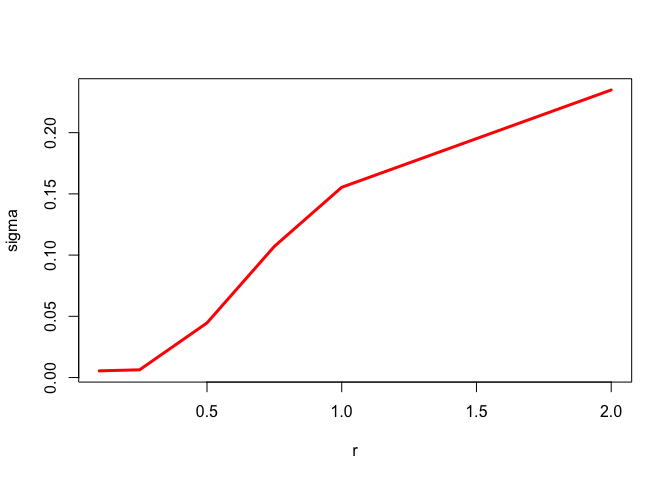Figure 3: De Gruijter Data: rStress

We put the nuber of iterations and the rStress values in a small table.

## r:  0.10  iterations:    29103  rStress:  0.005464
## r:  0.25  iterations:     3605  rStress:  0.006310
## r:  0.50  iterations:     3566  rStress:  0.044603
## r:  0.75  iterations:     3440  rStress:  0.107113
## r:  1.00  iterations:   100000  rStress:  0.155392
## r:  2.00  iterations:   100000  rStress:  0.234877
Figure 4 shows the six Shepard diagrams. We see the clustering for r = 2. For r = .1 the Shepard diagram becomes concave, indicating that the larger dissimilarities are underestimated and reflecting the fact that for small powers the powered distances will all be close to one.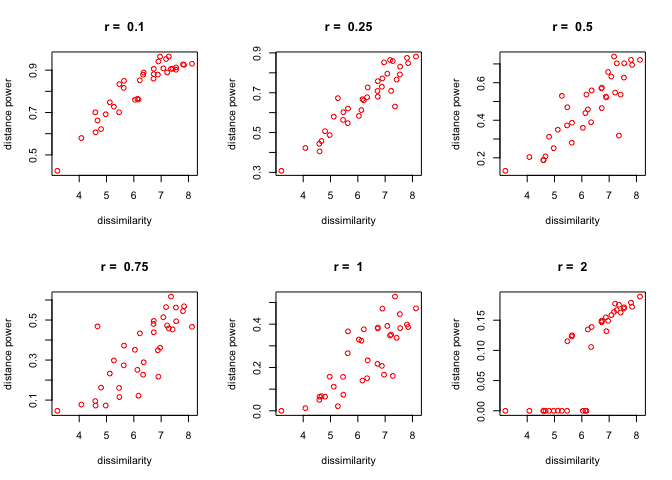Figure 4: De Gruijter Data: Shepard Diagrams

## 7.3 Ekman Color Data

We use the color data from Ekman (1954), with two dimensions and unit weights. As we know from previous analyses, MDS of the Ekman data gives a very good fit and a very clear representation of the color circle.

##      434  445  465  472  490  504  537  555  584  600  610  628  651
## 445 0.14
## 465 0.58 0.50
## 472 0.58 0.56 0.19
## 490 0.82 0.78 0.53 0.46
## 504 0.94 0.91 0.83 0.75 0.39
## 537 0.93 0.93 0.90 0.90 0.69 0.38
## 555 0.96 0.93 0.92 0.91 0.74 0.55 0.27
## 584 0.98 0.98 0.98 0.98 0.93 0.86 0.78 0.67
## 600 0.93 0.96 0.99 0.99 0.98 0.92 0.86 0.81 0.42
## 610 0.91 0.93 0.98 1.00 0.98 0.98 0.95 0.96 0.63 0.26
## 628 0.88 0.89 0.99 0.99 0.99 0.98 0.98 0.97 0.73 0.50 0.24
## 651 0.87 0.87 0.95 0.98 0.98 0.98 0.98 0.98 0.80 0.59 0.38 0.15
## 674 0.84 0.86 0.97 0.96 1.00 0.99 1.00 0.98 0.77 0.72 0.45 0.32 0.24
In our algorithm we always start with a classical metric scaling solution (Torgerson 1958). We minimize rStress for r equal to 0.1, 0.25, 0.5, 0.75, 1, 2. The six solutions are plotted jointly in Figure 5. Because of the very good fit, solutions for different values of r are similar and very much on the color circle.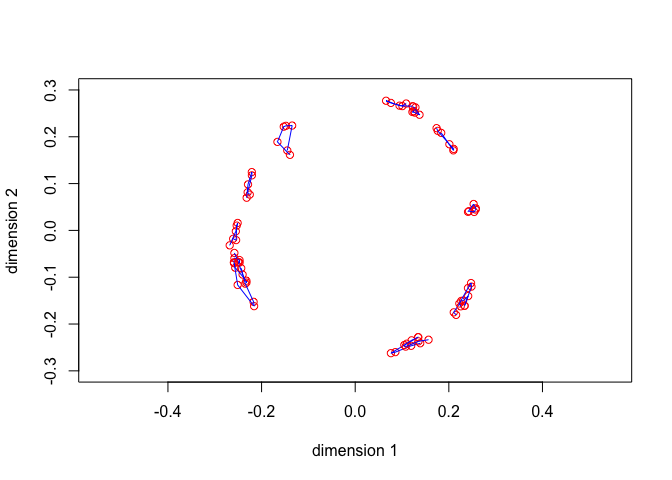Figure 5: Ekman Color Data: Configuration

The Shepard diagrams in figure 6 are interesting, because, unlike the configurations, they are quite different, becoming more and more convex as r increases. The plot for r = .25 looks best.